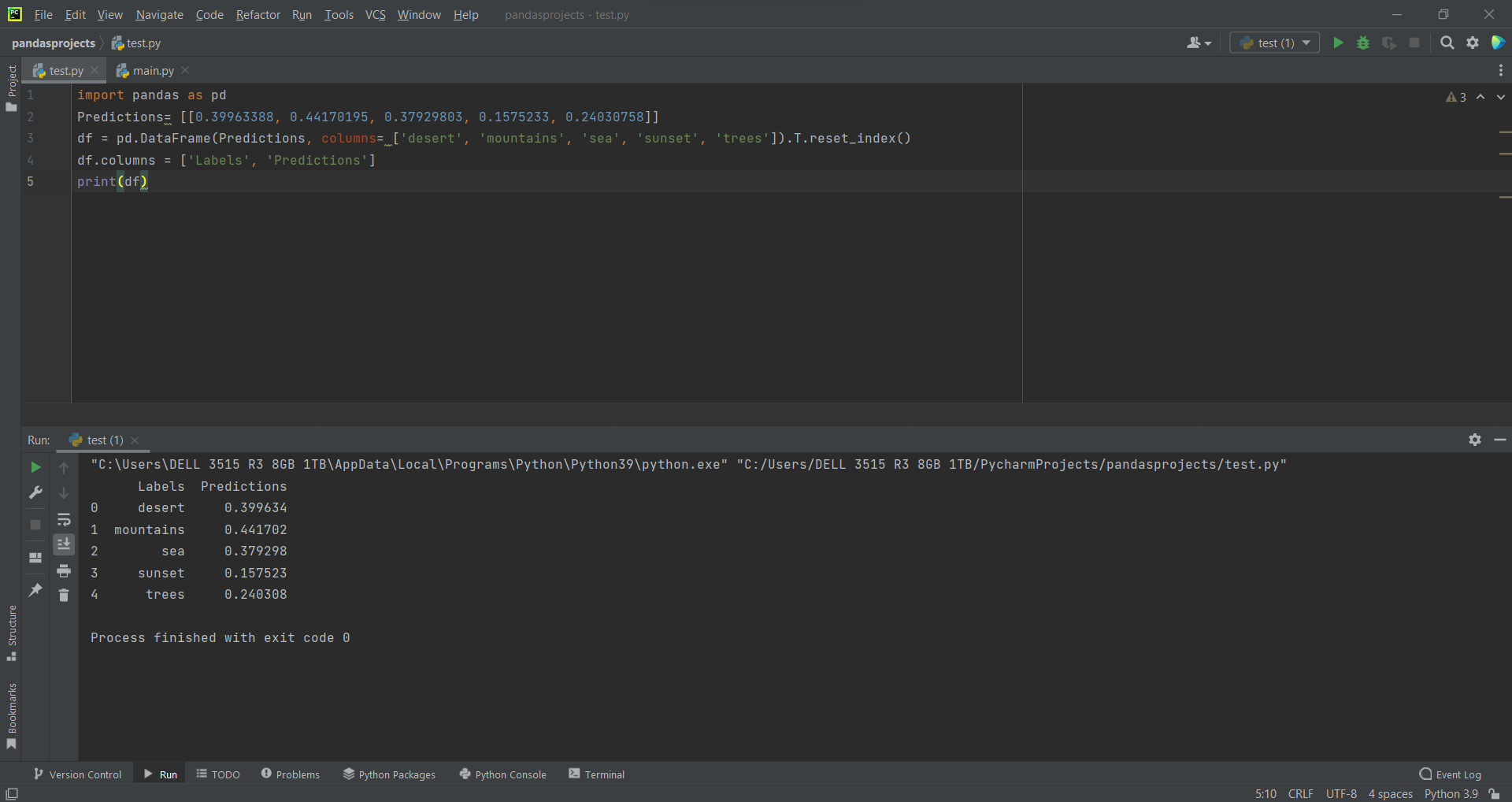# Fit Prediction Values in Panda Dataframeby Abdul Rawoof A RUpdated: Feb 21, 2023Solution Kit

In Python, to predict the labels of the data values based on the trained model predict() function is used, and the syntax for predict function is the model. predict(data). It accepts only a single argument, usually the data to be tested.

Predict function passes the input vector through the model and returns the output tensor for each data point, and since the last layer in your model is a single Dense neuron, then the output for any data point is a single value. We did not specify an activation for the last layer, and it will default to linear activation in Python. The predicted value is nothing but simulations that consider the estimation uncertainty and the fundamental uncertainty. For example, for any given of x, we go straight in the line and then move horizontally to the left of the data to find the value of Y. The predicted value of Y is denoted Y'. Dependent variables are those we want to predict, whereas independent variables are variables we use to predict the other variable's value.

Here is an example of how to implement fit prediction values in Pandas DataFrame:Fig : Preview of the output that you will get on running this code from your IDE.

### Code

In this solution we're using Pandas library.

### Instructions

Follow the steps carefully to get the output easily.

2. Copy the snippet using the 'copy' and paste it in your IDE.
3. Add required dependencies and import them in Python file.
4. Run the file to generate the output.

I hope you found this useful. I have added the link to dependent libraries, version information in the following sections.

I found this code snippet by searching for 'how to fit prediction values using panda dataframe' in kandi. You can try any such use case!

### Environment Tested

I tested this solution in the following versions. Be mindful of changes when working with other versions.

1. The solution is created in PyCharm 2021.3.
2. The solution is tested on Python 3.9.7.
3. Pandas version-v1.5.2.

Using this solution, we are able to fit predictions values using panda dataframe with simple steps. This process also facilities an easy way to use, hassle-free method to create a hands-on working version of code which would help us to fit predictions values using panda dataframe.

### Dependent Library

pandasby pandas-dev

Python37363Version:v2.0.0rc1Flexible and powerful data analysis / manipulation library for Python, providing labeled data structures similar to R data.frame objects, statistical functions, and much more

Support
Quality
Security
Reuse

pandasby pandas-dev

Python37363Version:v2.0.0rc1License: Permissive (BSD-3-Clause)

Flexible and powerful data analysis / manipulation library for Python, providing labeled data structures similar to R data.frame objects, statistical functions, and much more
Support
Quality
Security